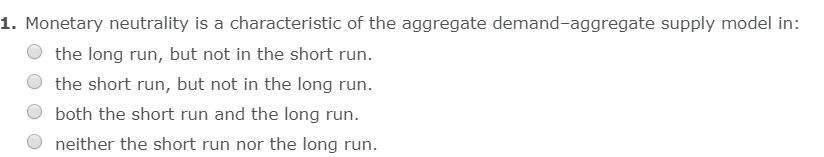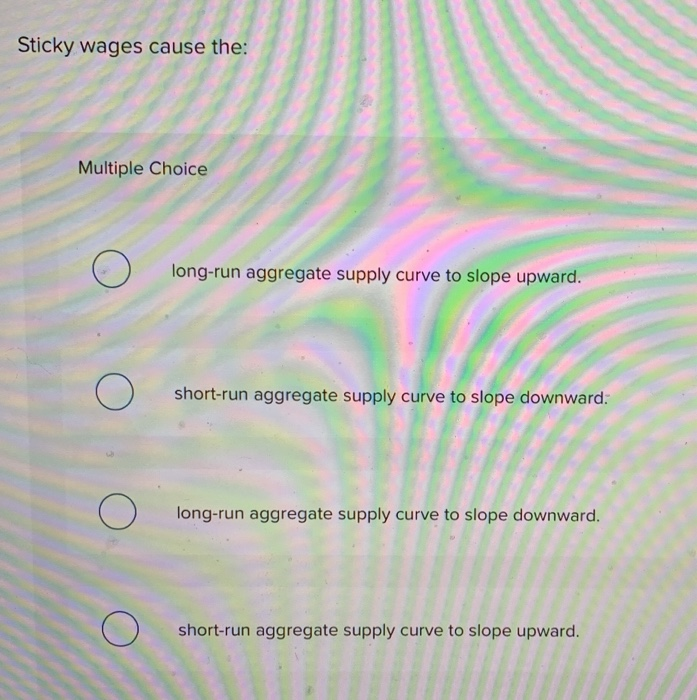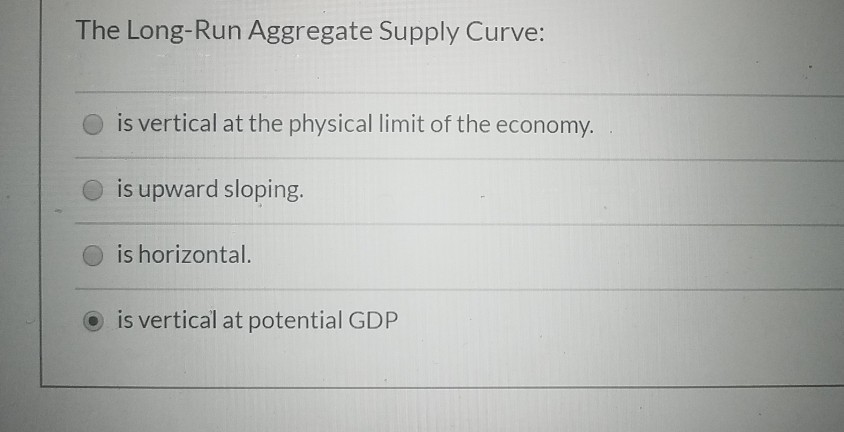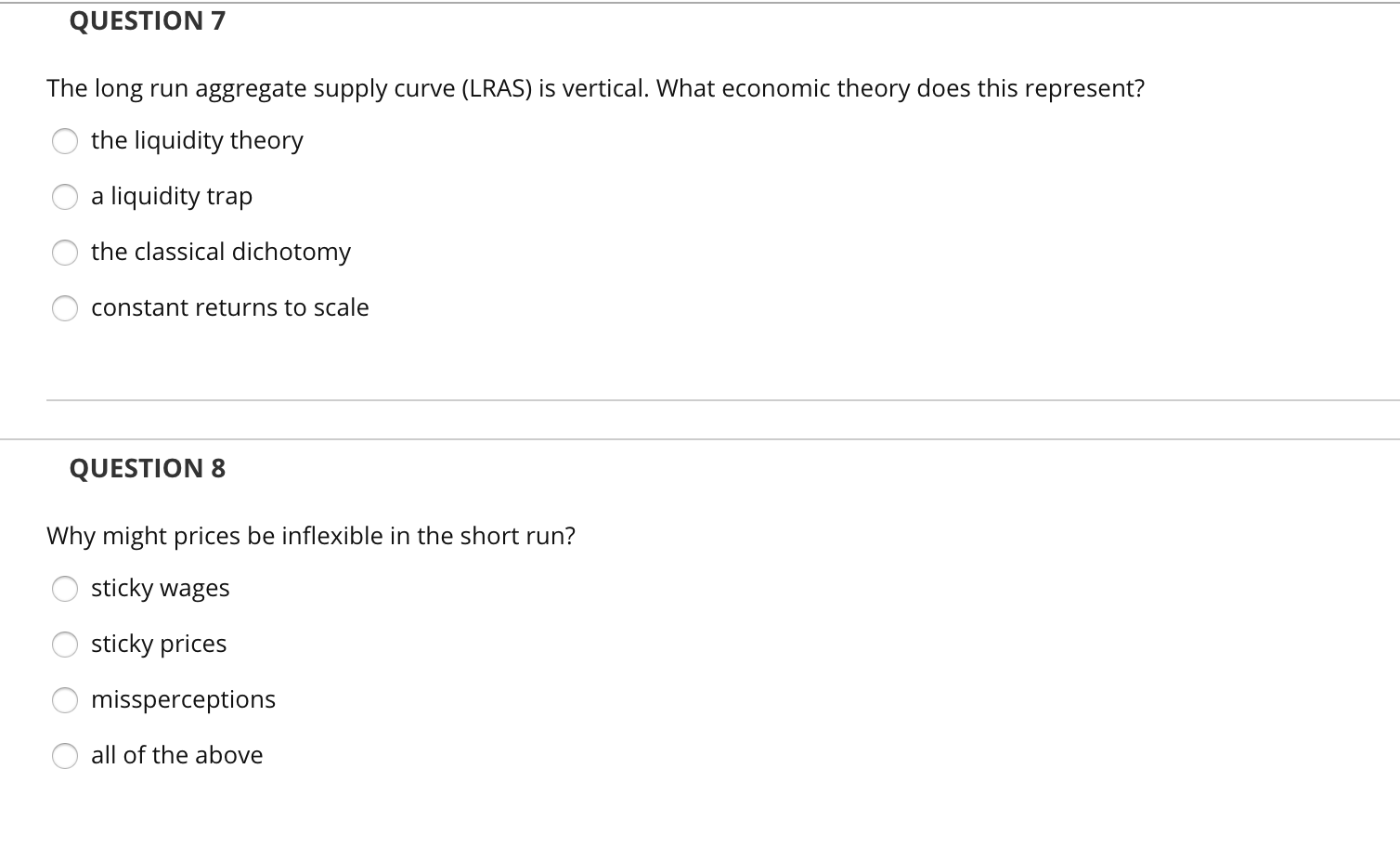Question

# The classical dichotomy and monetary neutrality are represented graphically by  an upward-sloping short-run aggregate-curve.  a vertical long-run aggregate-supply...

The classical dichotomy and monetary neutrality are represented graphically by
an upward-sloping short-run aggregate-curve.
a vertical long-run aggregate-supply curve.
an upward-sloping long-run aggregate-supply curve.
a downward-sloping aggregate-demand curve.

Vertical long run aggregate supply curve

Both theories indicate that monetary variables are not able to influence real variables in the long run because of Swift adjustment of the economy towards full employment. wages and prices are flexible so that any change in the money stock will proportionately change the price level so that real output remains unchanged.

#### Earn Coins

Coins can be redeemed for fabulous gifts.

Similar Homework Help Questions
• ### The long-run aggregate supply curve Group of answer choices is a graphical representation of the classical...

The long-run aggregate supply curve Group of answer choices is a graphical representation of the classical dichotomy. indicates monetary neutrality in the long run. is vertical All of the above are correct.

• ### Describe the​ short-run aggregate supply ​(SRAS​) curve and the​ long-run aggregate supply ​(LRAS​) curve. A. the...

Describe the​ short-run aggregate supply ​(SRAS​) curve and the​ long-run aggregate supply ​(LRAS​) curve. A. the SRAS curve is horizontal and the LRAS curve is upward sloping B. the SRAS curve is horizontal and the LRAS curve is vertical C. the SRAS curve is vertical and the LRAS curve is horizontal D. the SRAS curve is vertical and the LRAS curve is upward sloping Why is the​ short-run aggregate supply curve​ horizontal? A. because output is fixed in the short...

• ### 1. Monetary neutrality is a characteristic of the aggregate demand-aggregate supply model in: the long run,...1. Monetary neutrality is a characteristic of the aggregate demand-aggregate supply model in: the long run, but not in the short run. the short run, but not in the long run. both the short run and the long run. neither the short run nor the long run.

• ### Sticky wages cause the: Multiple Choice long-run aggregate supply curve to slope upward. short-run aggregate supply...Sticky wages cause the: Multiple Choice long-run aggregate supply curve to slope upward. short-run aggregate supply curve to slope downward. long-run aggregate supply curve to slope downward. short-run aggregate supply curve to slope upward.

• ### The Long-Run Aggregate Supply Curve: is vertical at the physical limit of the economy. is upward...The Long-Run Aggregate Supply Curve: is vertical at the physical limit of the economy. is upward sloping. is horizontal. is vertical at potential GDP

• ### Given a downward-sloping aggregate demand (AD) curve and an upward-sloping short-run aggregate supply curve (SRAS), equilibrium...

Given a downward-sloping aggregate demand (AD) curve and an upward-sloping short-run aggregate supply curve (SRAS), equilibrium occurs where the two intersect. The value on the vertical axis is the equilibrium price level and the value on the horizontal axis is the equilibrium value of real GDP or output. What happens to the economy when AD shifts? It is useful to sketch a graph and show the shift. Suppose, for example, interest rates fall or wealth increases due to a stock...

• ### 17- Both the long run and short run aggregate supply curve will shift when an event...

17- Both the long run and short run aggregate supply curve will shift when an event occurs which is expected to last only a short period of time. they are both upward sloping. a war occurs in the Middle East. the endowments of the factors of production changes 19- Cost-push inflation occurs when the aggregate supply curve shifts to the right, while aggregate demand remains stable. when the aggregate demand curve shifts to the left, while aggregate supply remains stable....

• ### In the aggregate demand and aggregate supply model, a. the factors that cause the individual supply...

In the aggregate demand and aggregate supply model, a. the factors that cause the individual supply curve to slope upward are the same as the factors that cause the short-run aggregate supply curve to slope upward. b. the upward-sloping short-run aggregate supply curve intersects the downward-sloping aggregate demand curve to determine the economy's price level and GDP. c. the factors that cause the individual demand curve to slope downward are the same as the factors that cause the aggregate demand...

• ### QUESTION 7 The long run aggregate supply curve (LRAS) is vertical. What economic theory does this...QUESTION 7 The long run aggregate supply curve (LRAS) is vertical. What economic theory does this represent? o the liquidity theory O a liquidity trap O the classical dichotomy O constant returns to scale QUESTION 8 Why might prices be inflexible in the short run? O sticky wages O sticky prices O missperceptions o all of the above

• ### The aggregate supply curve in the very short run is most likely: 1. Upward-sloping because wages...

The aggregate supply curve in the very short run is most likely: 1. Upward-sloping because wages and prices of other inputs do not fully adjust to changes in the price level. 2. Flat because output can be adjusted to a certain degree without a corresponding change in prices. 3. Vertical because wages and prices of other inputs fully adjust to changes in the price level.